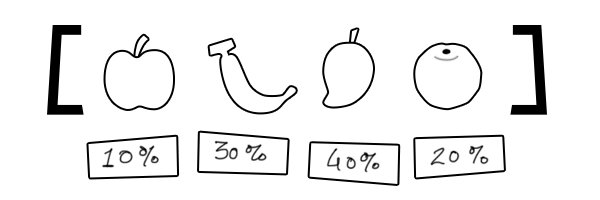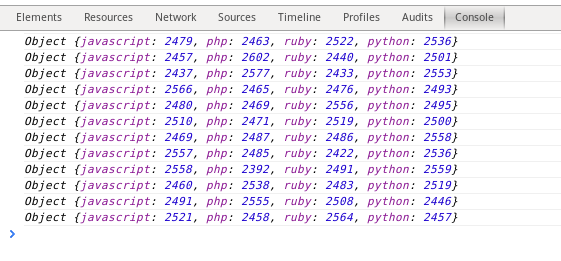# Weighted/Biased Random Number Generation with JavaScript based on ProbabilityFirst of all what is weighted random ? Let’s say you have a list of items and you want to pick one of them randomly. Doing this seems easy as all that’s required is to write a little function that generates a random index referring to one of the items in the list. Sometimes plain randomness is not enough, we want random results that are biased or based on some probability. This is where a simple weighted random generation algorithm can be used.

What's the one thing every developer wants? More screens! Enhance your coding experience with an external monitor to increase screen real estate.

## Implementation

Let’s write a quick simple implementation in JS. We need a set of random items to work with first. Let’s consider an array of programming languages.

```var lang = ['javascript', 'php', 'ruby', 'python'];
```

We may not prefer each language equally, so depending upon our abstract magnitude of preference we can assign a weight to each of them –

```var weight = [0.5, 0.2, 0.2, 0.1];
```

So we have 4 numbers for each language respectively, `0.5` (50%) for javascript, `0.2` (20%) for PHP, `0.2` (20%) for ruby and `0.1` (10%) for python. These numbers we assign are the probability or the weights that’ll define the random item picked from the `lang` array. If you notice all the weights add up to 1 or 100% which is the highest probability. Now it may not be super necessary to have all your weights be equal to 1 or similar, but this approach just seems more legit.

### Method 1

In order for the languages to be picked respective percent of the time, i.e., making them conform to the weights what we’d do is something simple. Basically repeat the items 10x or even 100x times based on the numbers we have. So let’s say we’re repeating 10x times, this is the list we’ll end up with –

```var weighed_list = [
'javascript', 'javascript', 'javascript', 'javascript', 'javascript',
'php', 'php',
'ruby', 'ruby'
'python'
];
```

Hopefully you get the idea! Usually 10x is probably fine, but with more items I believe a 100x multiplier seems better, which is what I do. So let’s make a function that’d accept both the arrays and produce the resulting array.

```var generateWeighedList = function(list, weight) {
var weighed_list = [];

// Loop over weights
for (var i = 0; i < weight.length; i++) {
var multiples = weight[i] * 100;

// Loop over the list of items
for (var j = 0; j < multiples; j++) {
weighed_list.push(list[i]);
}
}

return weighed_list;
};

var list = ['javascript', 'php', 'ruby', 'python'];
var weight = [0.5, 0.2, 0.2, 0.1];
var weighed_list = generateWeighedList(list, weight);

console.log(weighed_list.length);
```

Loop over the weights, multiply the weight by `100` and then in an inner loop duplicate the pertaining item that many times inside the `weighed_list` that’ll end up having a length of 100. That’s all we do, simple! Given we have lots of duplicate items, it’s more likely that the randomness will be affected as per our needs.

Next we need to write some code to generate a random number between `0` and `weighed_list.length` that can be used as the index to reference a biased random item.

```var rand = function(min, max) {
return Math.floor(Math.random() * (max - min + 1)) + min;
};

var random_num = rand(0, weighed_list.length);
console.log(random_num);
```

This random number generation code has been taken from here, feel free to read it for a good amount of explanation. Now call it to get a random number to be used as index to pick an item from our weighted list.

```console.log( weighed_list[rand(0, weighed_list.length)] );
```

That’s all, here’s the full piece of code –

```var rand = function(min, max) {
return Math.floor(Math.random() * (max - min + 1)) + min;
};

var generateWeighedList = function(list, weight) {
var weighed_list = [];

// Loop over weights
for (var i = 0; i < weight.length; i++) {
var multiples = weight[i] * 100;

// Loop over the list of items
for (var j = 0; j < multiples; j++) {
weighed_list.push(list[i]);
}
}

return weighed_list;
};

var list = ['javascript', 'php', 'ruby', 'python'];
var weight = [0.5, 0.2, 0.2, 0.1];
var weighed_list = generateWeighedList(list, weight);

var random_num = rand(0, weighed_list.length-1);

console.log(weighed_list[random_num]);
```

The random item picked will definitely be affected by the weights.

### Method 2

Having gone through the first approach which is fairly intuitive, let’s take a look at another one which is even more easier to grasp and implement. The process for this approach is something like this –

1. Sum up all the weights. In our case it’s going to be `0.5 + 0.2 + 0.2 + 0.1 = 1.0`.
2. Get a random number between `0` and the total weight (`1.0`).
3. If the random number falls in the first slot which is `0.5` or `weight` then choose the first item from the list. If it falls in the second slot which is between `weight` and `weight + weight` then select the corresponding item from the list. For third item random number must fall in the third slot which would be between `weight + weight` and `weight + weight + weight`, and the sequence goes on.

Let’s take a look at some code now.

```var rand = function(min, max) {
return Math.random() * (max - min) + min;
};

var getRandomItem = function(list, weight) {
var total_weight = weight.reduce(function (prev, cur, i, arr) {
return prev + cur;
});

var random_num = rand(0, total_weight);
var weight_sum = 0;
//console.log(random_num)

for (var i = 0; i < list.length; i++) {
weight_sum += weight[i];
weight_sum = +weight_sum.toFixed(2);

if (random_num <= weight_sum) {
return list[i];
}
}

// end of function
};

var list = ['javascript', 'php', 'ruby', 'python'];
var weight = [0.5, 0.2, 0.2, 0.1];
var random_item = getRandomItem(list, weight);

console.log(random_item);
```

First of all the `rand` function has changed, it’s the first version from the reference now so that we get numbers with fractional parts to work with, not just integers.

Next up, in the `getRandomItem` method we get the total weight by simply summing up all the weights using the Array.prototype.reduce method. Inside the loop we keep on summing up the weight from the first index of `weight` array and check whether the random number is less than or equal to the resultant weight or not. In the steps above when I was referring to the pertaining weight slots for the random number it might have seemed something hard to implement but now as you can see it’s pretty easy. So as the loop iterates the values and conditions inside are something like this –

1. `weight_sum = 0 + 0.5;` Check if `random_num <= 0.5`
2. `weight_sum = 0.5 + 0.2;` Check if `random_num <= 0.7`
3. `weight_sum = 0.7 + 0.2;` Check if `random_num <= 0.9`
4. `weight_sum = 0.9 + 0.1;` Check if `random_num <= 1.0`

If any of the conditions evaluate to true the respective list item will be returned.

## Experimenting

Wouldn't it be fun if we picked items randomly 1000 times and actually see what's the count percentage of each ? So I gave it a go using the first method described above.

```var random_check = {
javascript: 0,
php: 0,
ruby: 0,
python: 0
};

var random_num
, item;

for (var i = 0; i < 10000; i++) {
random_num = rand(0, weighed_list.length-1);
item = weighed_list[random_num];
++random_check[item];
}

console.log(random_check);
```

Nothing too fancy, just an object with the languages as properties with numeric values that gets incremented whenever respective random item is picked. Running them a few times, these are the results we get -Almost near to what we want! awesome sauce! Test it yourself if you want to. Incidentally, this is the results without the algorithm -Here's the testcase for the second method that yields similar results as the first one.

## Conclusion

We developed these methods during our HTML5 game development process where we needed some objects to appear randomly on screen but based on some probability or weights so that we could control the randomness to make the gameplay much better and fun. Although this method can be applied to pretty much any application where you're not happy with the uniform randomness of the existing generating methods.

Recommended from our users: Dynamic Network Monitoring from WhatsUp Gold from IPSwitch. Free Download## Author: Rishabh

Rishabh is a full stack web and mobile developer from India. Follow me on Twitter.

## 5 thoughts on “Weighted/Biased Random Number Generation with JavaScript based on Probability”

1.kikito says:

I recently had to do something similar to your second problem. If you store your “intervals” as an ever-increasing array of numbers, you can use quicksort to find the right the interval to which the random number belongs to a bit more efficiently. Obviously this solution will start paying off once you have a large enough dataset.

1.ekelleyv says:

While this may be fine for smaller lists, it is not even close to the most efficient solution. As kikito mentioned, you could do an efficient search through the ordered array of cumulative random number cutoffs to get it down to O(log(n)) lookup time. However, using an alias method, you can get lookup time down to O(1). Here is the paper on Vose’s method

2.asdf says:
``````
var choices = ['apple', 'bannana', 'peach', 'pear'];
var weights = [0.4, 0.3, 0.2, 0.1];

var thresholds = [], weight;
while(weight = weights.shift())
thresholds.push({threshold: (!thresholds.length ? weight : thresholds.reduce(function(el, sum){ return sum + el; }) + weight), item: choices.shift()});

function getWeightedlyRandomChoice() {
var rand = Math.random();
for(var i = 0; i < thresholds.length; i++)
if(rand < thresholds[i].threshold)
return thresholds[i].item;
}

console.log(getWeightedlyRandomChoice());
``````
3.North Pole Labs says:

Great post Rishabh! I’ve been using the second method for a few projects, and kikito makes a good point. I have to say the result set from method 1 is pretty awesome, I’ll give it a try sometime. The link to Vose’s method posted in another comment seems to be down, so here is another link to it for anyone else that runs across this.

4.BlueRaja says:

Keep going, you’re almost there!

This method is good, but it is slow when there are many items/weights. You can extend it in two ways:
1. Use a tree instead of a list. Then choosing a random item, or adding/removing items/weights from the list, are all O(log n) operations, instead of O(n) like they are now.
2. Construct a table. Constructing the table is non-trivial and O(n) (you use the ‘Walker Alias Method’, see here), but once it’s constructed items can be chosen in O(1). So, adding/removing items will be as slow as your implementation, but choosing a random item will be significantly faster.

I’ve implemented both of these algorithms in a C# library here.